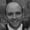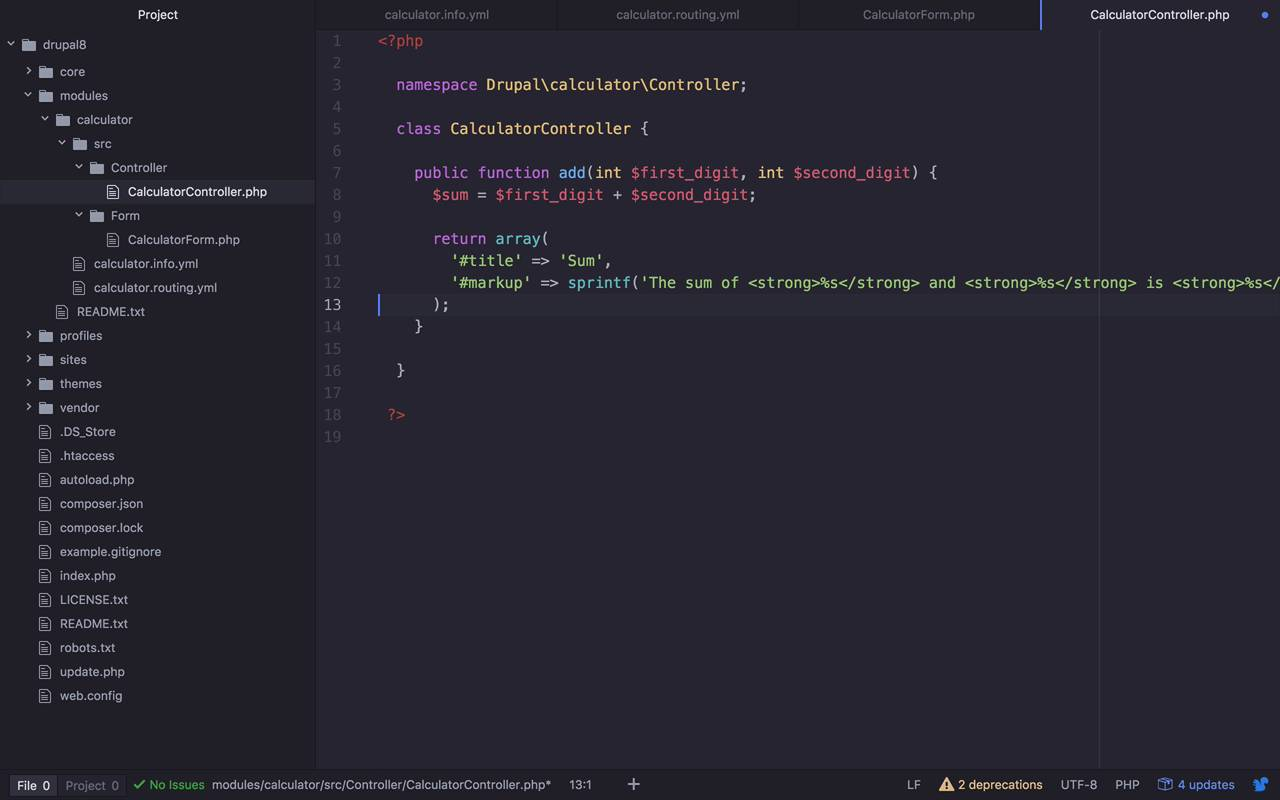FREELessons: 13Length: 1.4 hours• Overview
• Transcript

# 3.2 Implement the Add Operation

With our form ready to go, it's time to add some server-side processing so we can handle the mathematical operations that our calculator exposes. In this lesson, we will start with addition and create a PHP method to handle it.

## 4.Conclusion1 lesson, 02:31

### 3.2 Implement the Add Operation

Now, I'm gonna show you a fairly simplistic way how you can implement the functionality for the different operations, by showing you an example of the addition option. And we're gonna follow that through from beginning to end on how to get the data, understand that it's an add operation, and then submit it to an appropriate route that's going to handle that particular add operation. Now, there's a couple different ways that you could solve this problem. You could create a single function that's going to take in the different operators and the operand. And it can figure it out in there, or it can use separate routes. Whatever you really wanna do is fine. I'm gonna go the separate route version, and kinda follow along that path. But once again you can feel free to tailor this any way that you would like. So really what I'd like to do at this point is once I submit the form, I wanna understand what type of operation the user wants to do is an add, subtract, multiply, or delete. And then from that point, I want to send that data to the appropriate route and then have the route handle the rest. So the way that we're going to do that first off is we're going to create specific routes. Now, I'm just gonna start with the add, and then I'll let you kind of fill in the rest. But I'm gonna show you how I'm going to handle this. So, I'm going to create another route and I'm gonna call it calculator_add. My path is going to be my/calculator/add, and then it's gonna have two values in here. It is going to have first digit and the second digit. So in order for me to do that, I am going to say first_digit, and then I'll do another slash, and I will do the second digit, second_digit like that. So this how I'm gonna format the URL for this particular path once again, or you can change it and have it be anything that you want. But I think this should be sufficient for what I'm trying to do. And then we are going to create our controller once again. Remember we need a fully qualified domain name. I'm just going to copy this and then make some slight modifications, this is not in Form, this is going to be in Controller. And this is not CalculatorForm, this is going to be CalculatorController, and the operation is going to be add, just like that. So let's go ahead and save this. So what my logic is going to be, once I submit the form, if I notice that this is an add operation, I am going to get those two values, first digit and second digit. And then I'm going to set the redirect on my form state just like I did before to go to this particular route. Passing those values and then this calculator controller over here will handle that. So let's go ahead and do that part next. So I'll come to my calculator controller, and that means I need to come in here and I need to define a public function of add. And this is gonna take in two values. A first digit, and this is going to be an integer. An integer and this is going to be first_digit. And then we're gonna take in a second parameter which is going to be second_digit, just like that. And all at this case I really wanna do, is I wanna get the sum of these two, so I can say the sum is going to be equal to, and I can say the first digit plus the second digit. So now I have the sum, and I want to simply return a new array. And within this array, remember I can return a couple of different things. I'm going to return the title, and this title is simply going to be, in this case it's gonna be sum, because I'm doing an addition operation. And then I'm going to do mark up in this case. And we'll just do some fairly simplistic markup, kind of like I did before. In this case, I will say the sum of. And then we'll do some more strong stuff in here again. And I'm gonna do this a couple of times. Let's go ahead and copy this. So I'm gonna say the sum of, and then here I'm gonna put the first digit, eventually. And then I'm gonna put in here another value, which is gonna be the second_digit. And then I'll say is and then I will have, at the end here I will make this be the sum. Something like that. So I think that should be fine. So now I need to pass in all of these values. So I need to pass in first_digit, I need to pass in second_digit. And I also need to pass in sum, just like that. Okay, so now I can save this. So now in my public function add, I'm taking in two digits, first and second. I'm going to calculate the sum, because that's what this particular operation wants to do. Then I'm going to return an array that's gonna have a title of Sum, so you'll see Sum on the output there. And then you'll also see my markup here, the sum of whatever these values are. So let's go ahead and save this. Make sure my routing is saved here. So I can test this out a little bit. So let's come to performance. Let's clear all caches. Let's come back over here to my calculator. And if I were to say add and do 1/1, what I should see here is the sum of 1 and 1 is 2. Which is great, now I can add these numbers together, but I want my form to drive this. I don't want the user to have to enter this information into my route. So we're just going to tie these things together now in our form. So the way that we're gonna handle this is we're gonna come into submitForm. And the first thing that I wanna do is I want to get a hold of that operation. Because that's really what's driving everything here. So I'm going to say the op is gonna be equal to, remember we wanna go into our form state and then we wanna get a value, and what's the value we wanna get? We wanna get the value of operation right here. So we're going to say get the value of operation, and the value of that operation is gonna be one of these right here. Add, subtract, multiply or delete. So there's a couple different ways that we can handle that. We could do some if statements. I'm going to use a switch block here and I'm going to switch on op. And then I can come in here and I can say in this case if this is add, then all I really wanna do at this point is I want to forward this onto the appropriate location, and then I wanna pass in the appropriate route parameters. So all we need to do is kind of similarly to what we saw before, I'm going to do a form_state, a I'm going to set the redirect. And I want to go to the calculator_add route, and I need to pass a couple of values here. I wanna pass first digit, and first digit is going to be equal to and this case I need to get the values that I've submitted in my form here for first digit and second digit. So the way that we can do that actually is I'll do that up here. So I can save myself the typing later, so I'll say first digit is going to be equal to the form state. We're going to get our value of first digit like that. And then let's just copy this, and we'll go ahead and do the same thing for the second digit. Say second digit here, we'll copy that, we'll do second digit here, get rid of the dollar sign, save that. So now, we're going to pass in as the first digit, $first_digit, and we'll pass in for second_digit,$second_digit just like that. And put in a semicolon here, we'll save, come back in here. We don't have to worry too much about this other stuff right now, so let's go ahead and save that. So now, theoretically, once I show this form and I select the add operation, I should come down in here and I should be redirected to my calculator add location, my route. So let's go ahead and clear our caches again. And then we'll come in here, let's go back to our calculator. And we'll just refresh that, make sure we're clean. Okay, so let's go ahead and put in some digits. Let's say we did 100, or let's say 1,000, and we added to that maybe 2,000. If I hit submit, then I go to my add route, add 1,000 and 2,000. And I get the sum of 1,000 and 2,000 is 3,000. Well there you go. You have now implemented from beginning to end a very simple calculator that can complete an addition operation. And really if you wanted to extend this and go any further than this, you should be able to see how simple it would be to simply add in some additional routes to handle subtract, multiply, and divide. And simply add in a little additional logic into your form to handle those operations. And similarly, into the calculator controller. So at this point, I will let you go ahead and pause the videos, or take a little bit of a break. And spend a little bit of time implementing those particular operations and see if you can tie all the pieces together. And then when you come back, I'll show you my implementation, and you can see how I would have gone about solving those other operations as well.

Back to the top